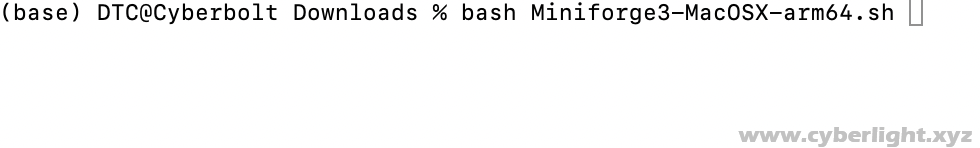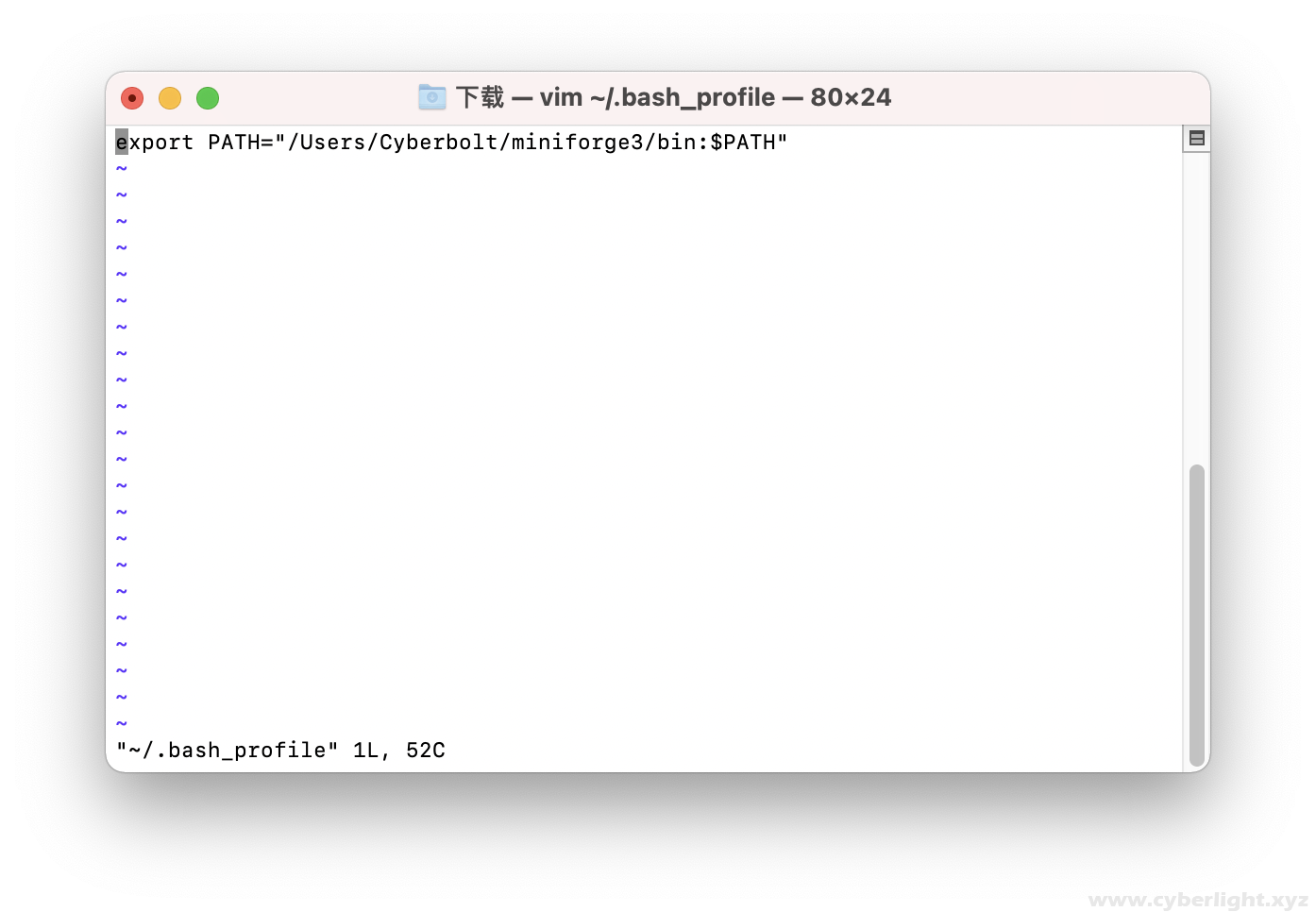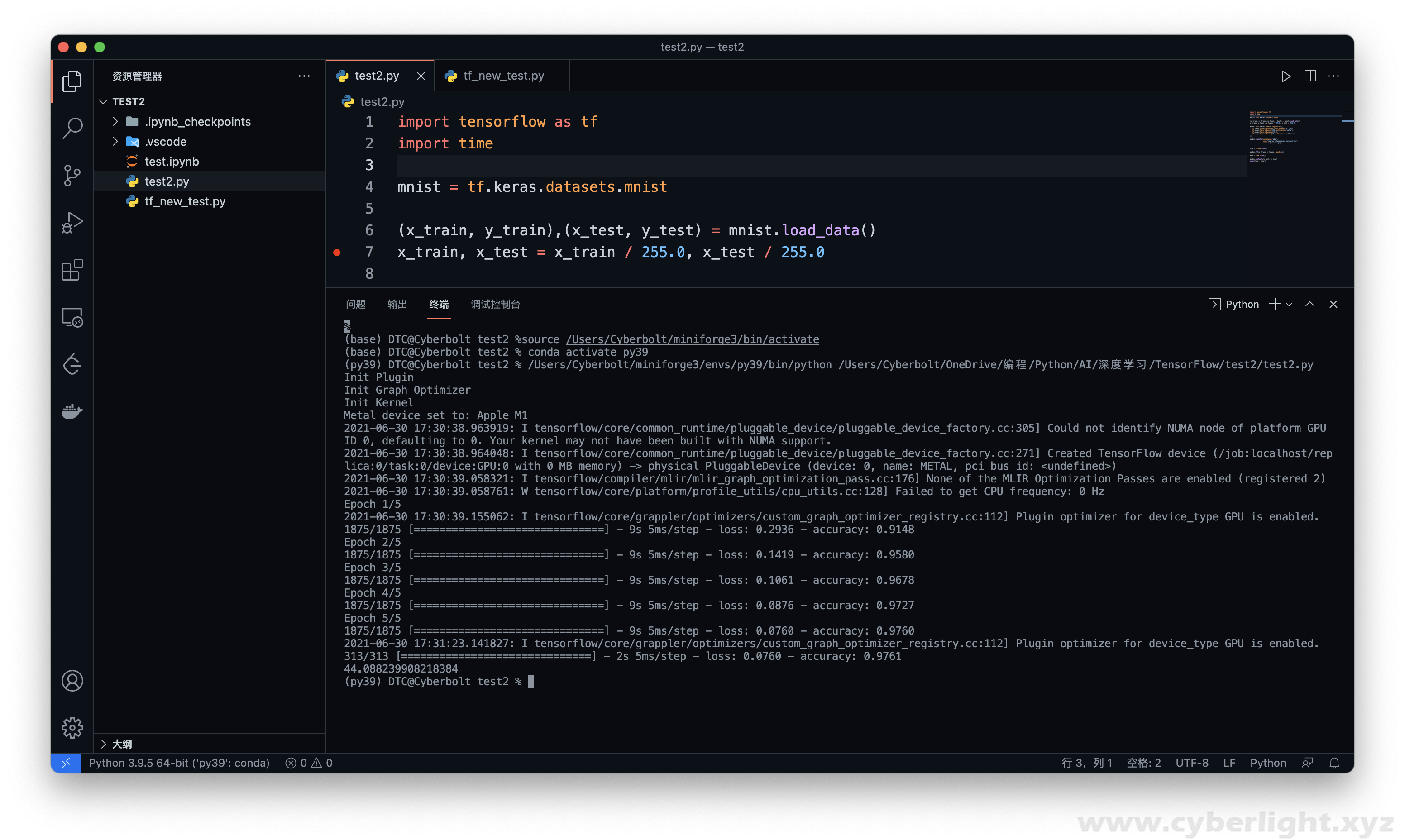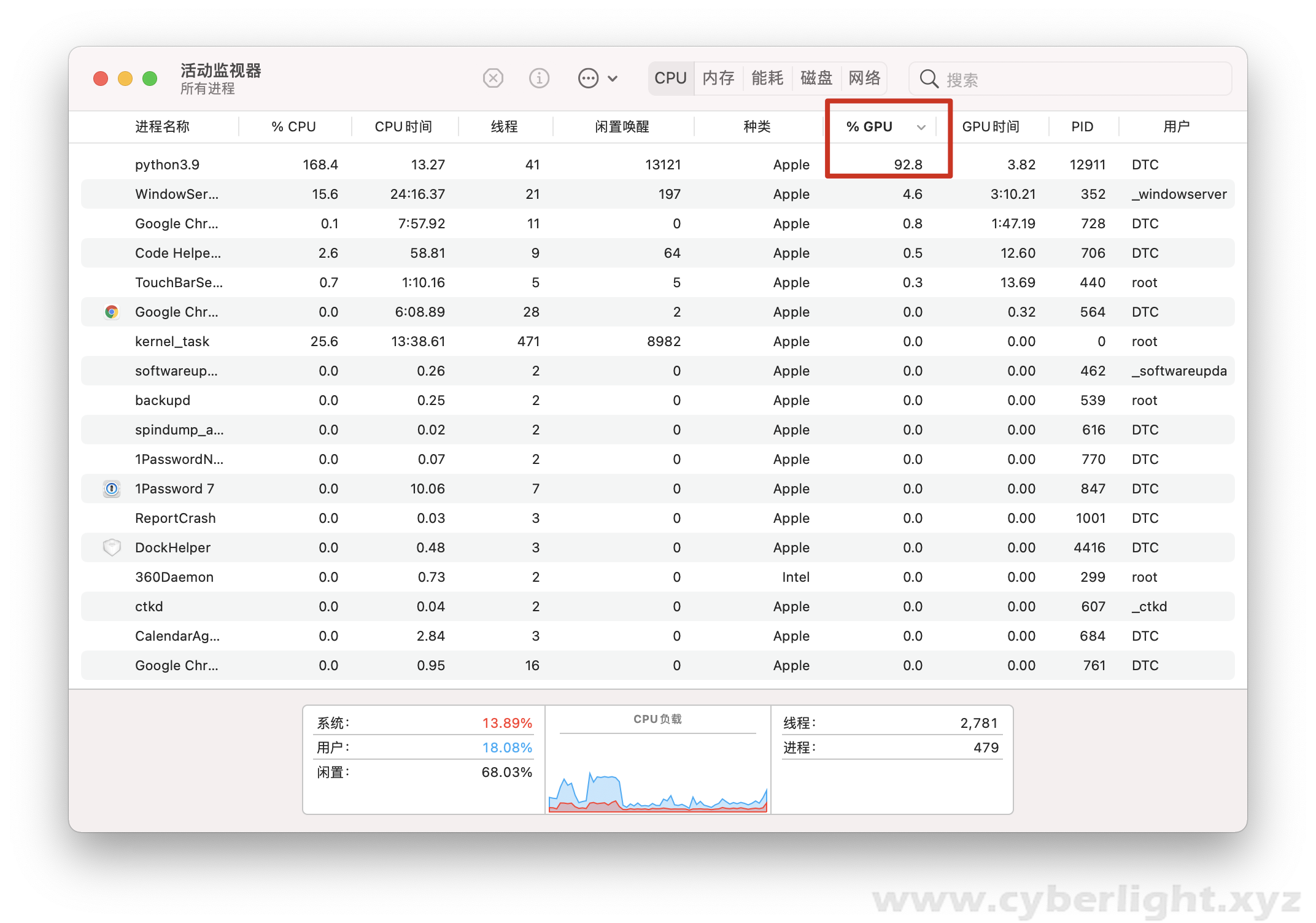# MacBook Pro M1 搭建 TensorFlow 2.5 深度学习环境（支持 Mac GPU 加速）

2021-06-30 17:40:48   Cyberbolt``bash Miniforge3-MacOSX-arm64.sh````vim ~/.bash_profile``

``export PATH="/Users/Cyberbolt/miniforge3/bin:\$PATH"````source \$HOME/.bash_profile````conda create -n py39 python=3.9``

``conda activate py39````````conda install -c apple tensorflow-deps
python -m pip install tensorflow-macos
python -m pip install tensorflow-metal``````

``````import tensorflow as tf
import time

mnist = tf.keras.datasets.mnist

x_train, x_test = x_train / 255.0, x_test / 255.0

model = tf.keras.models.Sequential([
tf.keras.layers.Flatten(input_shape=(28, 28)),
tf.keras.layers.Dense(128, activation='relu'),
tf.keras.layers.Dropout(0.2),
tf.keras.layers.Dense(10, activation='softmax')
])

loss='sparse_categorical_crossentropy',
metrics=['accuracy'])

start = time.time()

model.fit(x_train, y_train, epochs=5)

end = time.time()

model.evaluate(x_test, y_test)
print(end - start)``````